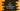# C# program to iterate over the characters of a string## C# program to iterate over the characters of a string:

In this post, we will learn how to iterate over the characters of a string one by one. We will write one program that will iterate over the characters in different ways. Mainly, we need to write a loop that will iterate through the characters. We can do it in many ways. We can use a for loop, while loop, do-while loop or foreach to solve this. Let’s take a look at these methods one by one.

### Method 1: By using a for loop:

We can do this by using a for loop. First, we will get the size of the string using string.Length and using a for loop, we will iterate through the characters of the string one by one. string[i] is used to access the character at index i.

Below is the complete program:

``````using System;
class Program {
static void Main() {

string given_str = "Hello World";
int size = given_str.Length;

for(int i = 0; i < size; i++){
Console.WriteLine(given_str[i]);
}
}
}``````

Here,

• give_str is the string to print the characters.
• size is an integer variable to hold the length of this string. .Length is used to get the length.
• The for loop runs from i = 0 to i = size -1, i.e. it runs for each index of the characters in the string.
• Inside the for loop, we are printing the characters for each index that is pointed by i.

### Method 2: By using a while loop:

This can be also done by using a while loop. Below is the complete program:

``````using System;
class Program {
static void Main() {

string given_str = "Hello World";
int size = given_str.Length;
int i = 0;

while(i < size){
Console.WriteLine(given_str[i]);
i++;
}
}
}``````

It is same as the above program. Here, we initialized one variable i as 0 and the while loop runs from i = 0 to i = size - 1, i.e. exactly same as the for loop. Inside the loop, it is incrementing the value of i, but for loop was doing it in the first condition statement.

### Method 3: Using a do-while loop:

It is same as the while loop. The only difference is that it executes the do block instead of while.

Below is the complete program:

``````using System;
class Program {
static void Main() {

string given_str = "Hello World";
int size = given_str.Length;
int i = 0;

do{
Console.WriteLine(given_str[i]);
i++;
}while(i < size);
}
}``````

### Method 4: Using a foreach loop:

Using foreach, we can iterate through the string characters. It is similar to for loop. The only difference is that we don’t have to get the length of the string and iterate over the characters by using the length.

Below is the program:

``````using System;
class Program {
static void Main() {

string given_str = "Hello World";

foreach(char ch in given_str){
Console.WriteLine(ch);
}
}
}``````

Here, we are iterating through the characters one by one using the foreach loop. It will print similar output.